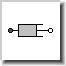Integer Product - MapleSim Help

Integer ProductProduct of Integers

 Description The Integer Product (or Product) component computes the product of the elements of the input vector. Note that the output is 0 if the input vector has zero length.
 Equations $y=\left\{\begin{array}{cc}\mathrm{Product}\left(u\right)& 0<{n}_{u}\\ 0& \mathrm{otherwise}\end{array}$

Connections

 Name Description Modelica ID $u$ Vector of Integer input signals u $y$ Integer output signal y

Parameters

 Name Default Units Description Modelica ID ${n}_{u}$ $2$ Number of input connections nu

 Modelica Standard Library The component described in this topic is from the Modelica Standard Library. To view the original documentation, which includes author and copyright information, click here.